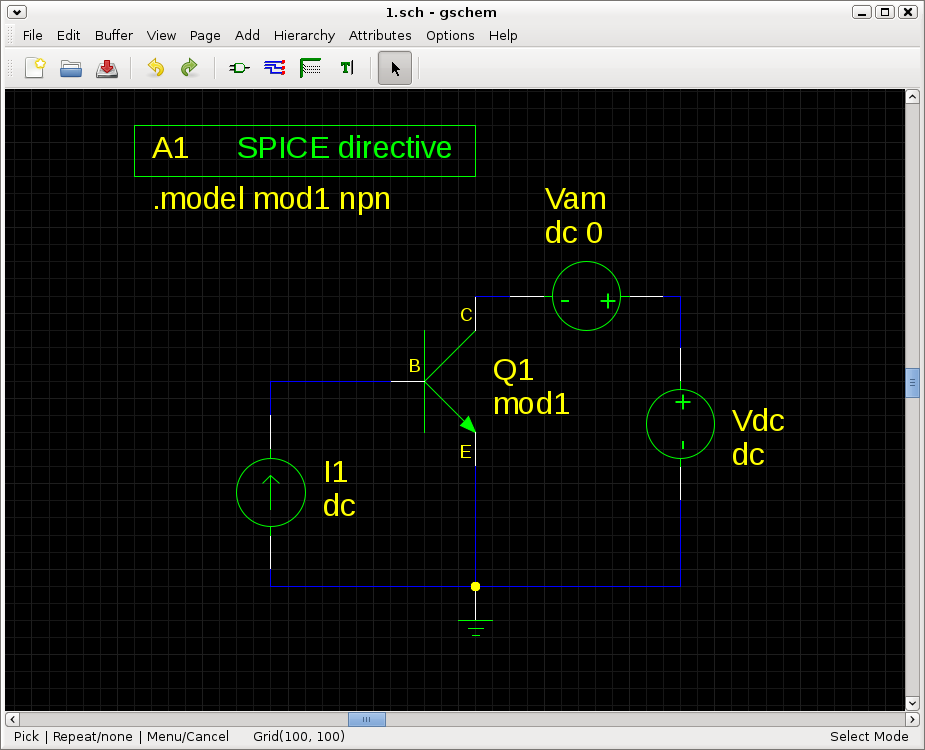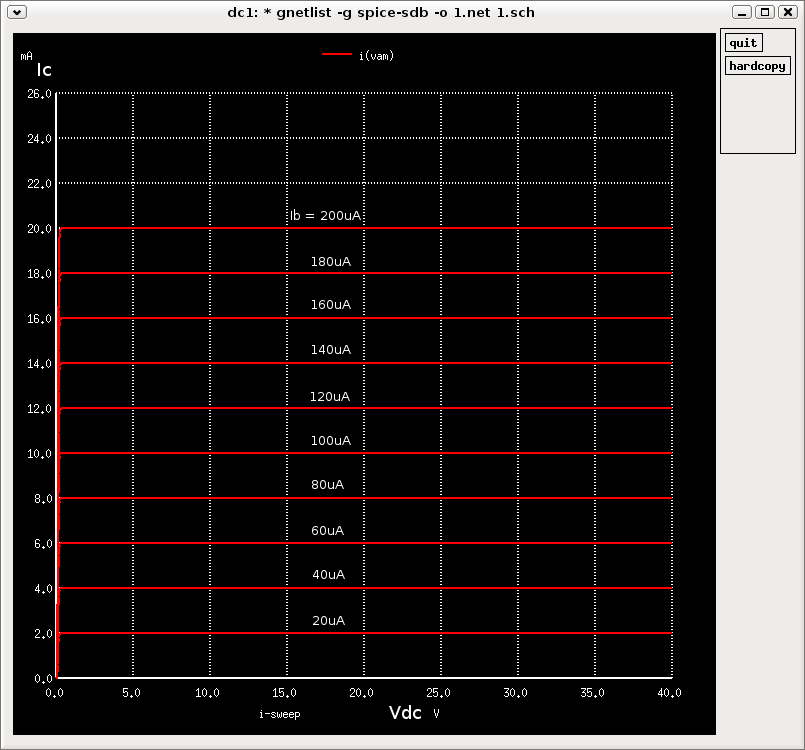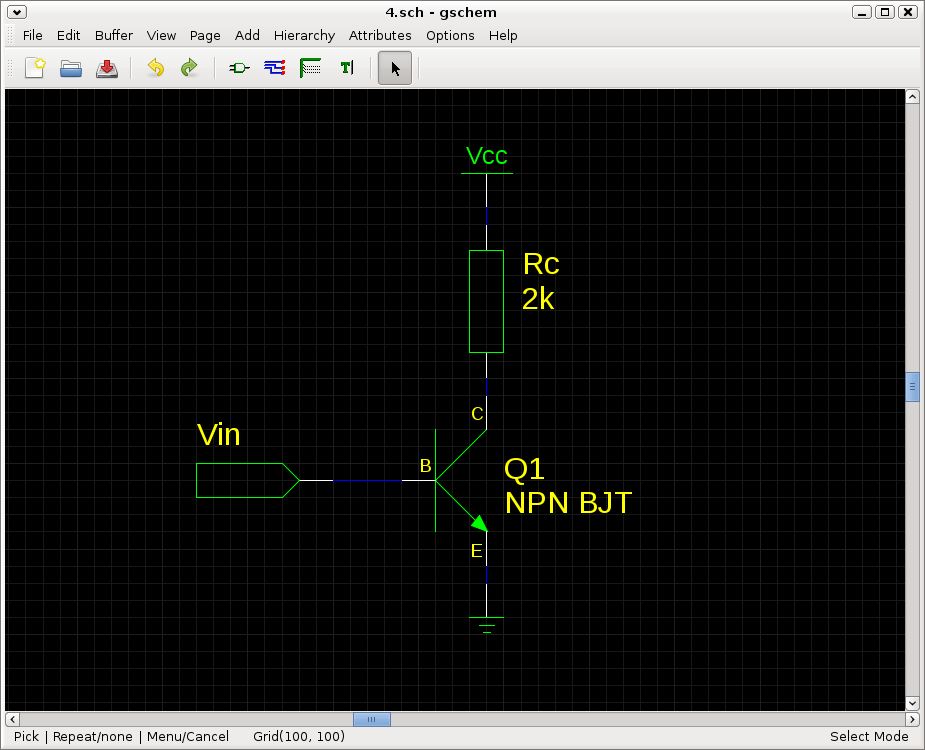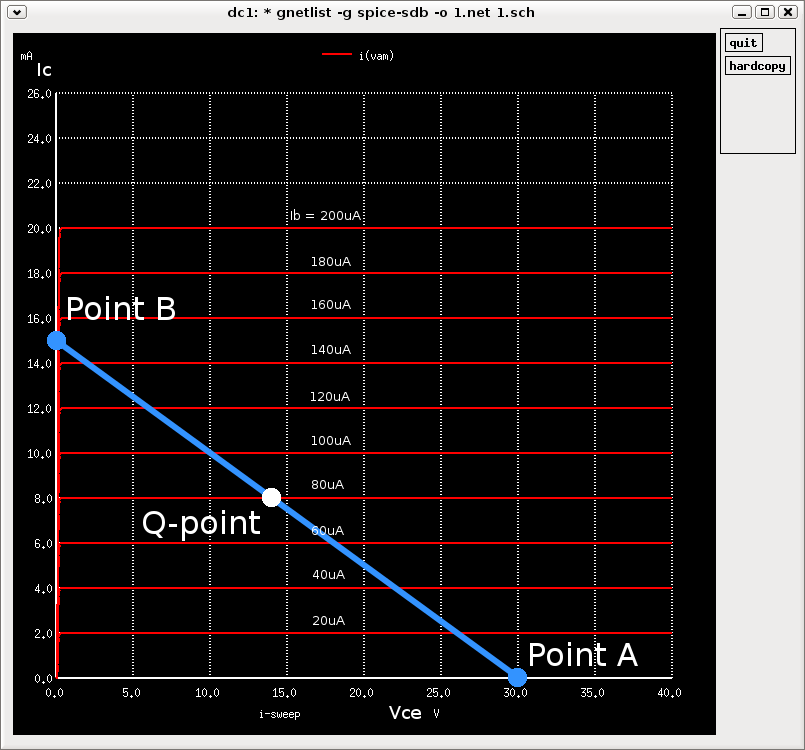Let's use this circuit to generate characteristic curves of a transistor:`dc vdc 0 40 0.02 i1 20u 200u 20u`
Performs a DC Analysis by sweeping the supply voltage Vdc from 0V to 40V in 0.02V increments and for each step varies I1 (the base current Ib) from 20uA to 200uA in 20uA increments (or it could be the other way round).

Then enter:
`plot i(vam) ylimit 0 25m`

`plot i(vam)` plots the collector current Ic (the current through the ammeter Vam) against supply voltage Vdc. `ylimit 0 25m` limits the y axis to a minimum of 0A and a maximum of 25mA:Using a supply voltage of 30V (Vcc) for example, you can draw a load line to find the Q-point (where the load line intersects the characteristic curve). Class A biasing would have a Q-point in the middle of the active (linear) region, approximately 15V. A good explanation of how to draw the load line can be found in Chapter 1.8 of this article.

Let's do an example; suppose you had a collector resistor Rc of 2k. At cutoff, no current (or negligible current) flows through the collector, so no current flows through resistor Rc, meaning there is no voltage dropped across Rc. The collector is at maximum potential: Vce = Vcc. We plot this point where Ic = 0A and Vce = Vcc (30V), let's call it Point A. At saturation, all the voltage is dropped across the resistor, so the collector is at 0V (or close to 0V). The current Ic is Vcc/Rc = 30/2k = 0.015A. We can plot the point where Ic = 0.015A and Vce = 0V (Point B). The load line is the line drawn between Point A and Point B (because of Ohm's Law being a linear equation and because of the axes of the graph being linear). Let's say we have DC biased the circuit to a base current Ib of 80uA, the Q-point would be where the load line intersects that particular characteristic curve:We can see that if we DC bias the base current to 120uA for example, the Q-point would be at just over 5V. This would not be in the middle of the linear region using this particular load value of Rc.

For a biasing tutorial, check out this link.